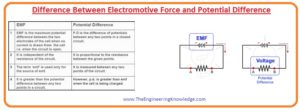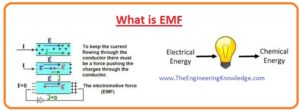Hello, friends, I hope you all are doing great. In today’s tutorial, we will discuss the Difference Between Electromotive Force and Potential Difference. The potential difference and EMF are types of energy. The basic difference between emf and a potential difference is that emf is generated when any other form of energy is transformed in the electrical energy while in potential difference electrical energy is transformed in the other type of energy.

In today’s post we will have a detailed look at both emf and potential difference compare them to find their differences. So let’s get started with Difference Between Electromotive Force and Potential Difference.

#### Difference Between Electromotive Force and Potential Difference

EMF

• The energy given to the one coulomb of charge is called EMF.
• It measured in Volts.
• Its symbolic representation is ε.
• The generation source of emf is dynamo or battery.
• Its value does not depend on the resistance of circuitry.
• EMF causes the current to flow in the circuit.
• The value of EMF is larger than the potential difference between 2 points.
• Its value remains constant.
• It also called the maximum value of voltage transferred by the battery.
• It generated in electrical, magnetic, and gravitational fields.
• Emf meter is used to find its value.

Potential Difference

• Potential difference is the energy used by the one coulomb of charge to move from one point to another.
• Its measuring unit is volt.
• Its symblic reprsentation is V.
• It is generated with the battery.
• Potential difference is a direct relation with the resistance of circuit,
• It can send a current between 2 points.
• When the battery is completely charged its value is less than the emf.
• A potential difference is generated in the magnetic field.
• It is measured with the voltmeter,#### What is Potential Difference

• The energy utilized by the one coulomb of charge to move from one point to another.
• Its measuring unit is volts and denoted with the V. Voltmeter is used to find value of potential difference.
• The formula of P.D is given as.

P.D=work/charge

#### What is EMF

• The EMF is the net voltage generated by the power source. In simple words the quantity of energy given by the supply source to every coulomb of charge.
• It also measured in volts and denoted with ε (epsilon).
• The extreme voltage obtained through the circuit is called emf. In real it is produced when fluctuation exits in the magnetic field.
• The formula of emf is given below.

EMF=work/charge

ε=w/q

• The EMF is a category of energy that moves the unit positive charge from the positive end of power source to a negative end.#### Comparison between EMF and Potential Difference

• The emf is energy provided to every coulomb of charge while P.D is a quantity of energy used by 1-coulomb charge.
• The EMF is denoted with the ε and V is a symbolic representation of potential difference,
• There is no dependency of EMF at the interior resistance of circuitry and P.D depends on the resistance of circuitry.
• The EMF measured the energy transmission in complete circuitry and P.D is a calculation of energy among 2 points of circuitry.
• In completely charged circuit emf is larger than the P.D and when the circuit is completely charged the value of P.D is equal to the EMF.
• The magnitude of emf is constant and the magnitude of P.D is not constant.
• The cause of the potential difference is EMF and P.D is the effect of p.D.
• When there is no current in the circuit emf exits in the circuit and when there is no P.D when no current flows in the circuitry.
• The extreme voltage delivered by the battery is EMF and P.D is less the maximum delivered voltage.

So friends it is a detailed post about the difference between emf and potential difference. If you have any further query ask in comments. See you in next post have a good day.